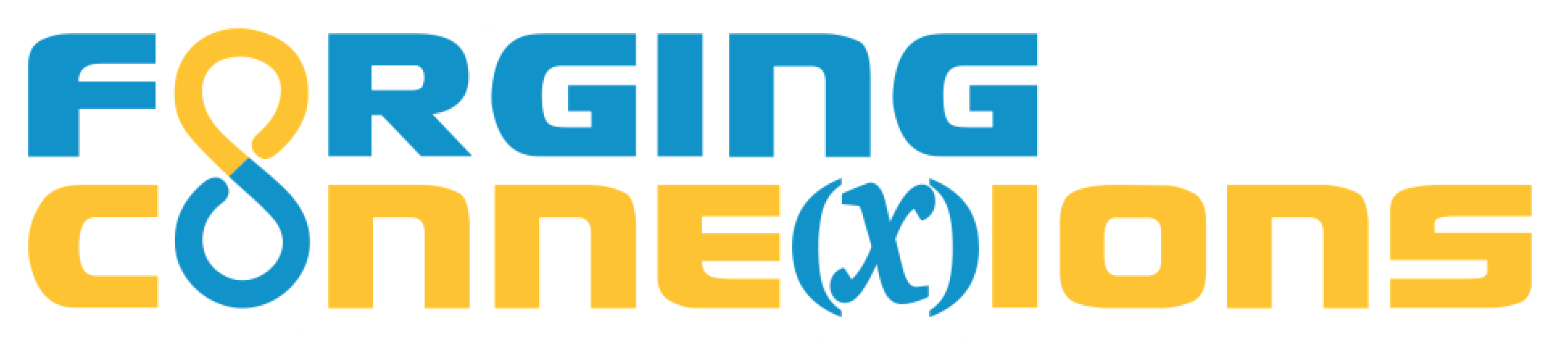2019 Regional Presentations with Web Sketchpad

2019 NCTM Regional Meeting

The SSS Theorem: Don’t Assume It, Prove It!

SSS is traditionally a postulate. By following CCSS and defining congruence via transformations, we can prove it. We’ll use Web Sketchpad tools to superpose one triangle on another, and capture the construction steps to create a proof of SSS. This and related lessons are freely available here: https://geometricfunctions.orgfc/present/pctm2019

Experience proving triangle congruence using a transformation approach. Using Web Sketchpad (WSP) technology, you’ll construct transformations that superpose one triangle on another. With WSP’s ability to capture your construction steps, the proof of the SSS Theorem is “transformed”! Bring a laptop or tablet to try free, classroom-ready lessons.

Not So Complex: A Geometric Approach to Complex Numbers from Addition through Euler’s Formula (April 2019)

Take your understanding of complex numbers to a new level using the dynamic visualization capabilities of Web Sketchpad. We’ll gain mathematical insights into complex number arithmetic, de Moivre’s theorem, and Euler’s formula through applying a geometric transformations approach to these topics.

• Presentations